## Boundary Tracing in Images

### Trace Boundaries of Objects in Images

This example shows how to trace the boundary of a single object and of all objects in a binary image.

```I = imread("coins.png"); imshow(I)```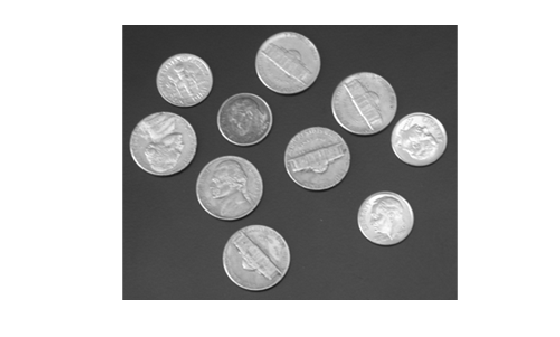Convert the image to a binary image. The `bwtraceboundary` and `bwboundaries` function work only with binary images.

```BW = imbinarize(I); imshow(BW)```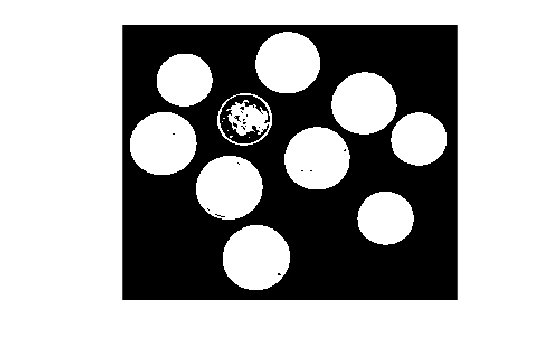Boundary of Single Object

To trace the boundary of a single object in the binary image, first determine the row and column coordinates of a pixel on the border of the object. For this example, select a column coordinate. The example then calculates the row coordinate of the topmost object in that column.

```numCols = size(BW,2); col =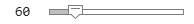60; row = find(BW(:,col),1)```
```row = 27 ```

To trace the boundary from the specified point, use the `bwtraceboundary` function. As required arguments, you must specify a binary image, the row and column coordinates of the starting point, and the direction of the first step. The example specifies north (`"N"`).

`boundary = bwtraceboundary(BW,[row, col],"N");`

Plot the border over the original grayscale image.

```imshow(I) hold on plot(boundary(:,2),boundary(:,1),"g",LineWidth=3);```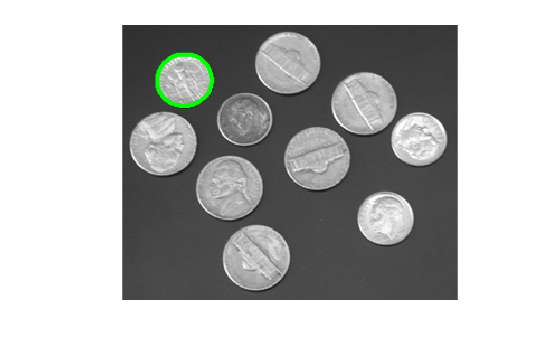Boundary of All Objects

In the binary image used in this example, some of the coins contain black areas that the `bwboundaries` function interprets as separate objects. To ensure that `bwboundaries` traces only the exterior of the coins, fill the area inside each coin by using the `imfill` function.

`BW_filled = imfill(BW,"holes");`

Trace the boundaries of all coins in the image by using the `bwboundaries` function. `bwboundaries` returns a cell array, where each cell contains the row and column coordinates for an object in the image.

`boundaries = bwboundaries(BW_filled);`

Plot the borders of all of the coins over the original grayscale image.

```for k=1:10 b = boundaries{k}; plot(b(:,2),b(:,1),"g",LineWidth=3); end```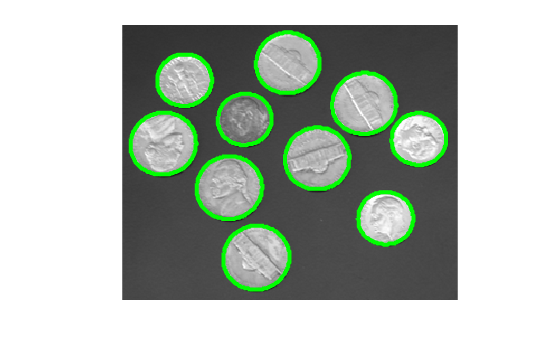### Select First Step Direction for Tracing

For certain objects, you must take care when selecting the border pixel you choose as the starting point and the direction you choose for the first step (such as north or south).

For example, if an object contains a hole and you select a pixel on a thin part of the object as the starting pixel, you can trace the outside border of the object or the inside border of the hole, depending on the direction you choose for the first step. For filled objects, the direction you select for the first step parameter is not as important.

To illustrate, this figure shows the pixels traced when the starting pixel is on a thin part of the object and the first step is set to north and south. The connectivity is the default value of 8.

Impact of First Step Direction on Boundary Tracing